Question

suppose X is a random variable best described by a uniform probability distribution with a=30 and...

suppose X is a random variable best described by a uniform probability distribution with a=30 and b=50 find p(30

The solution to this problem is given by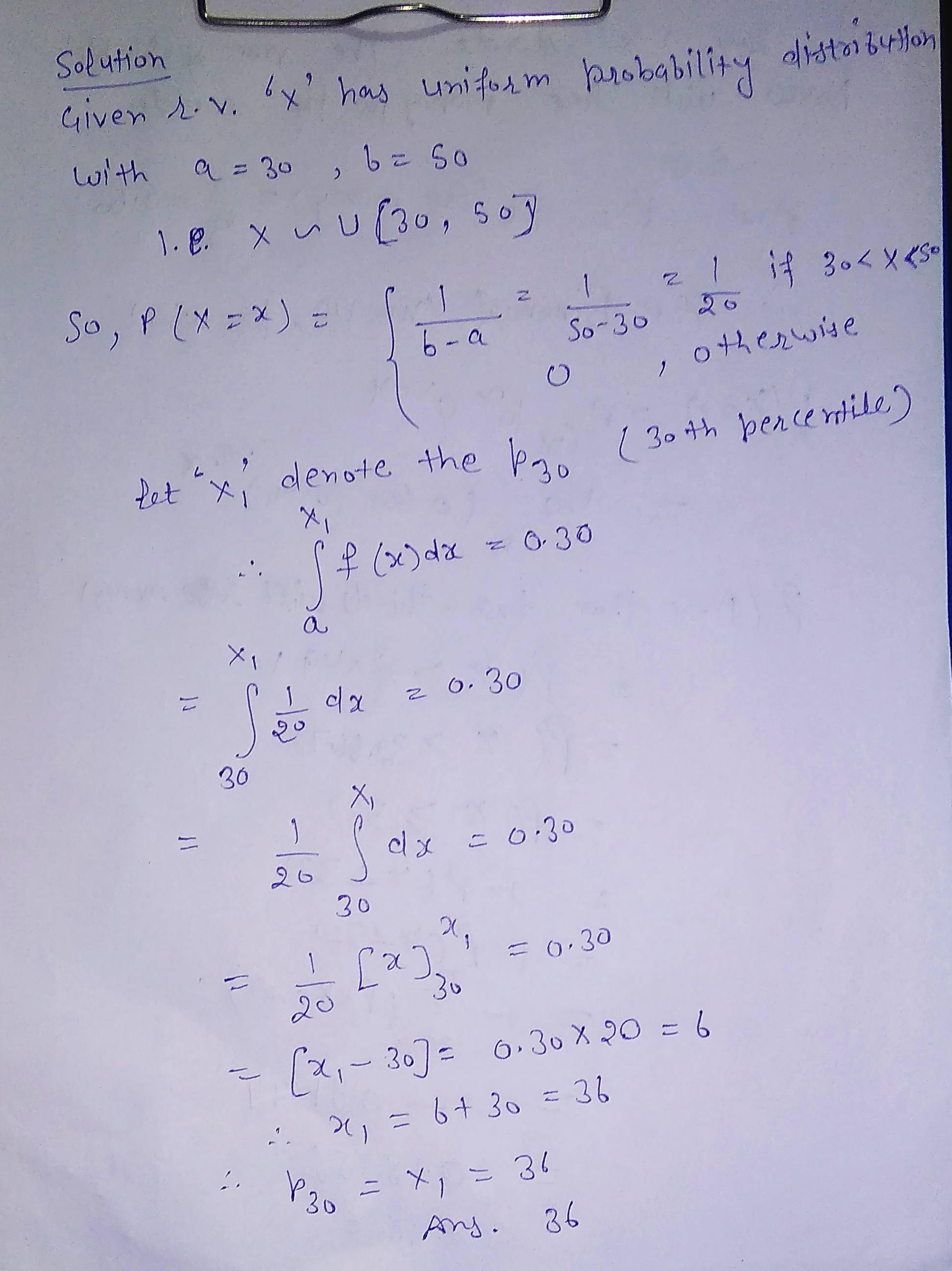Earn Coins

Coins can be redeemed for fabulous gifts.

Similar Homework Help Questions
• 1 point) Suppose a random variable x is best described by a uniform probability distribution with...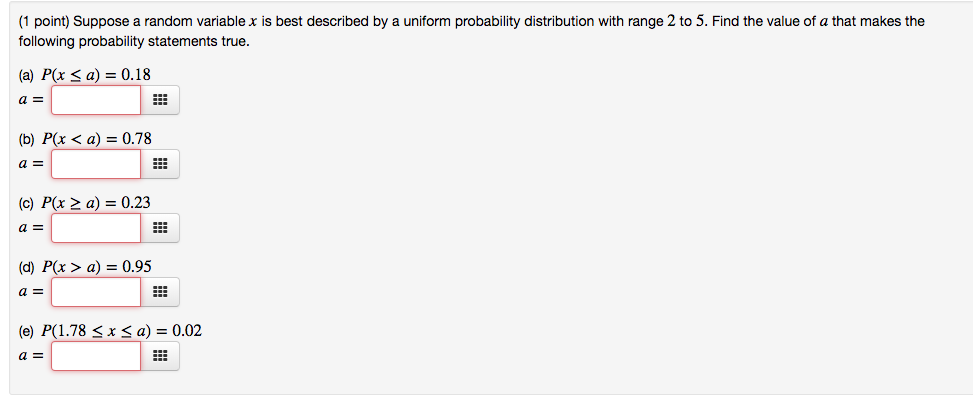1 point) Suppose a random variable x is best described by a uniform probability distribution with range 2 to 5. Find the value of a that makes the following probability statements true. (a) P(x <a) -0.18 a E (b) P(x < a) 0.78 (c) P(x 2 a) 0.23 (d) P(x > a) = 0.95 a= (e) P( 1.78 x a) = 0.02 a=

• that makes the following probability statements true. Suppose a random variable T is best described by...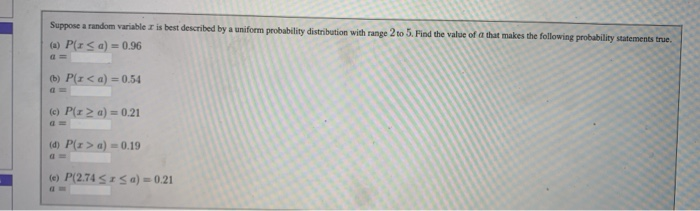that makes the following probability statements true. Suppose a random variable T is best described by a uniform probability distribution with range 2 to 5. Find the value of (a) PIS a) = 0.96 (b) Pr<a) = 0.54 (c) P(12 a) = 0.21 (d) P(x > a) = 0.19 (e) P(2.74 SIS a) = 0.21

• 4. (8 Marks) Suppose X is a random variable best described by a uniformly distribution or...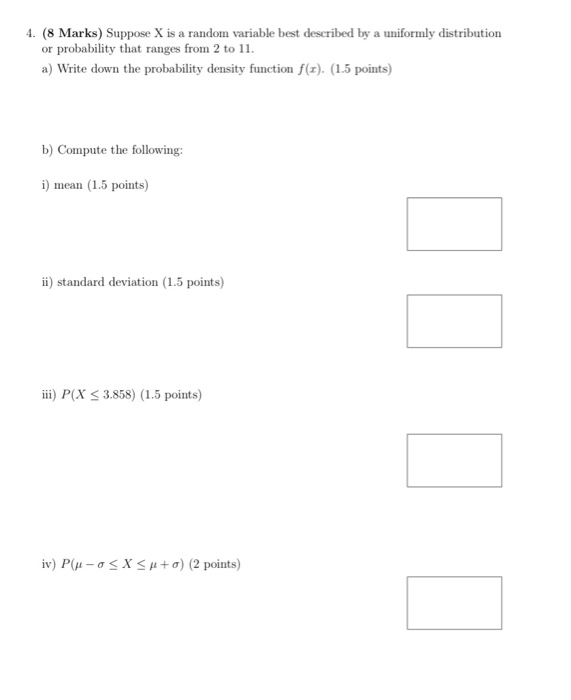4. (8 Marks) Suppose X is a random variable best described by a uniformly distribution or probability that ranges from 2 to 11. a) Write down the probability density function f(1). (1.5 points) b) Compute the following: i) mean (1.5 points) ii) standard deviation (1.5 points) iii) P(X < 3.858) (1.5 points) iv) P(-O< X <H+ o) (2 points)

• 18. Suppose the random variable x is best described by a normal distribution with u =...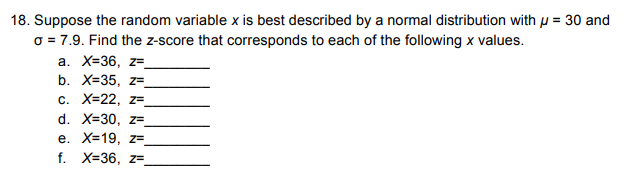18. Suppose the random variable x is best described by a normal distribution with u = 30 and o = 7.9. Find the Z-score that corresponds to each of the following x values. a. X=36, z= b. X=35, z=_ c. X=22, ze d. X=30, ZE e. X=19, ZF f. X=36, z

• Suppose x is a random variable best described by a uniform probability distrbution with c 10...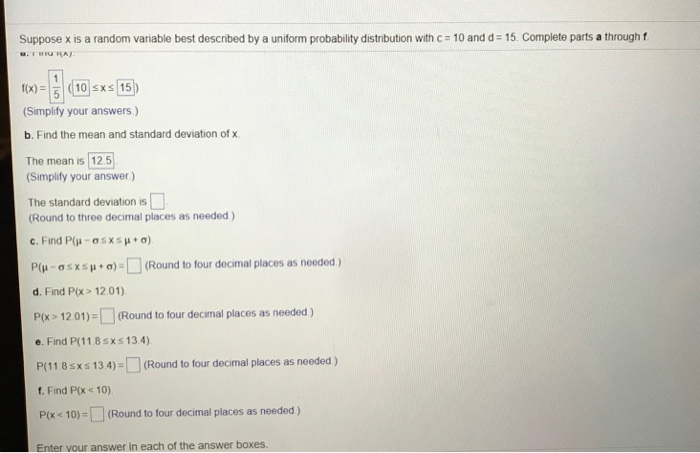Suppose x is a random variable best described by a uniform probability distrbution with c 10 and d 15. Complete parts a through f f(x)10 sxs 15 (Simplify your answers) b. Find the mean and standard deviation of x The mean is 125 Simplify your answer) The standard deviation is (Round to three decimal places as needed) P(u _ σ s x s μ + σ)-[ ] (Round to four decimal places as needed ) d. Find P(x> 12.01) P...

• Suppose a random variable x is best described by a normal distribution with = 60 and...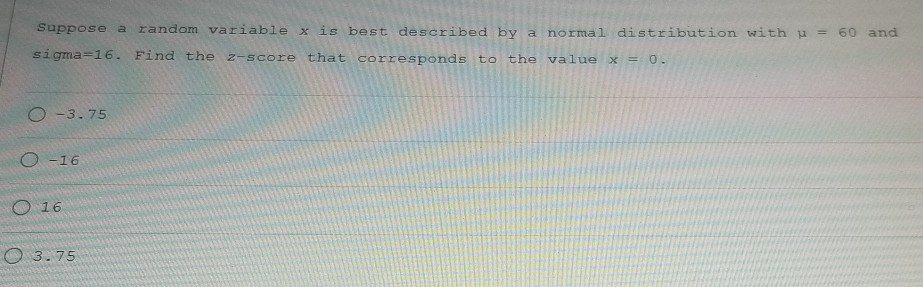Suppose a random variable x is best described by a normal distribution with = 60 and sigma=16. Find the z-score that corresponds to the value x = 0. 0 -3.75 0-16 O 16 0 3.75

• Suppose that X is a continuous random variable with probability distribution Suppose that X is a...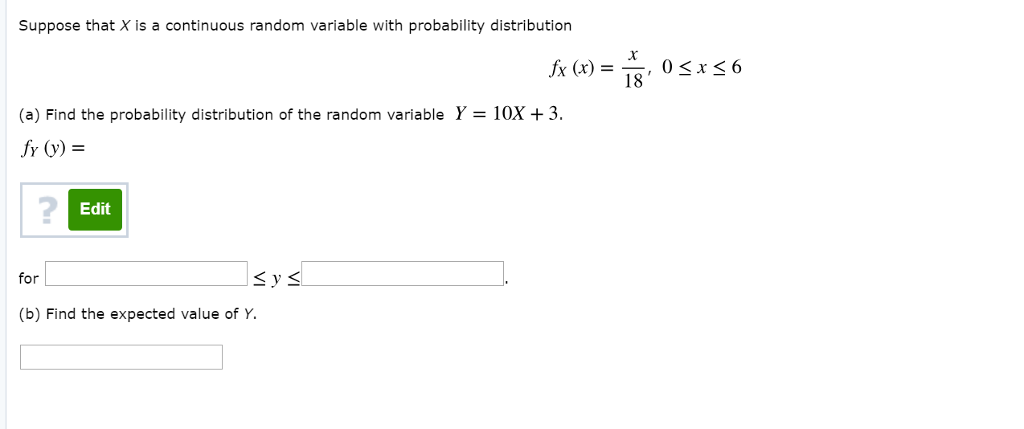Suppose that X is a continuous random variable with probability distribution Suppose that X is a continuous random variable with probability distribution O<x<6 18 (a) Find the probability distribution of the random variable Y-10X 3. fr o) 2 Edit for Sy s (b) Find the expected value of Y

• Question 19 4 pts Suppose a random variable x is best described by a normal distribution...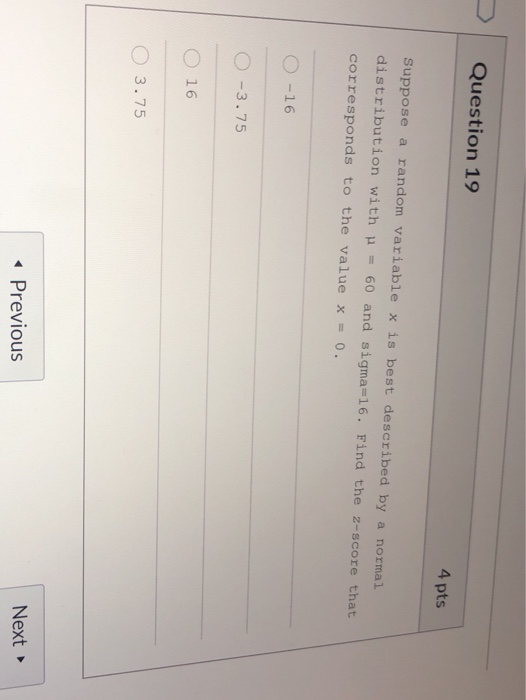Question 19 4 pts Suppose a random variable x is best described by a normal distribution with = 60 and sigma=16. Find the z-score that corresponds to the value x = 0. O-16 0 -3.75 16 O 3.75 Previous Next

• lo (P15) Suppose X is a random variable with the uniform distribution over the interval (1.2)...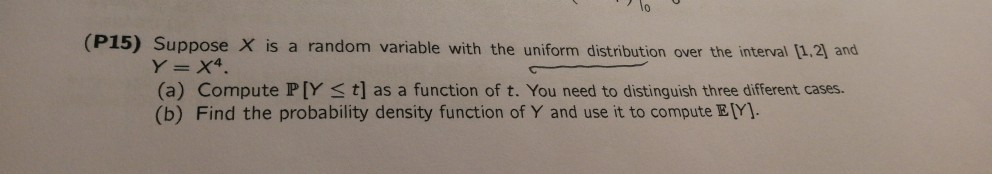lo (P15) Suppose X is a random variable with the uniform distribution over the interval (1.2) and Y = X4 (a) Compute P[Y St] as a function of t. You need to distinguish three different cases. (b) Find the probability density function of Y and use it to compute EY).

• Suppose that the random variable X has the discrete uniform distribution f(x) = { 1/4, r=...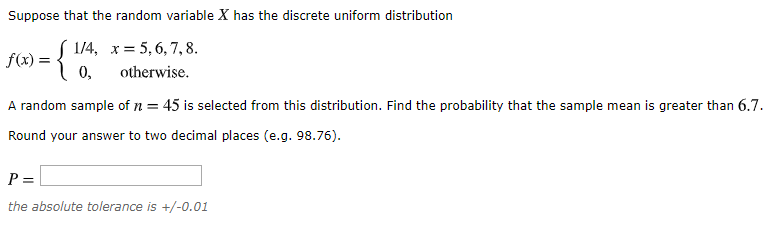Suppose that the random variable X has the discrete uniform distribution f(x) = { 1/4, r= 5, 6, 7, 8. 0, otherwise. A random sample of n = 45 is selected from this distribution. Find the probability that the sample mean is greater than 6.7. Round your answer to two decimal places (e.g. 98.76). P= the absolute tolerance is +/-0.01